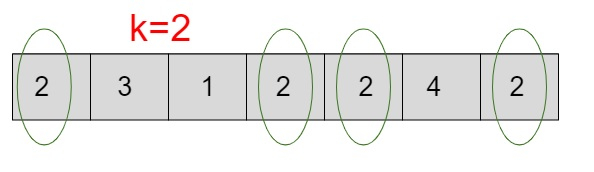# Probability of a key K present in array in C++

Given with an array of size ’n’ and the task is to find the probability of the given element k if available in an array.

Traverse the entire array till ‘n’ which is equals to the number of elements in an array and search for the given element or key ‘k’. If the element is present in an array than calculate its probability else print 0.

Input

arr[] = { 1, 2, 3, 4, 5, 6}
K = 5

Output

probability of a key 5 in an array is :0.166

Input

arr[] = { 1,2,3,4,5,6,7 }
K = 8

Output

probability of a key 5 in an array is :0

ExplanationGiven above is an array of size 7 and a key 2 so array will be traversed 7 times searching for a key value 2. Whenever 2 is identified increment a temporary variable let’s say counter by 1 and if the element is other than 2 move to next element without incrementing the counter. In the end −

• If the counter is 0 which means key is not present in an array than the probability will be 0

• If the counter is any value other than 0 than apply the formula to calculate the probability of key ‘’k’

Probability(k) = total number of ‘k’ occurrences / total number of elements

Total number of ‘K’ occurrence = 4

Total number of elements in an array = 7

Probability of key(k) = 4 / 7 = 0.57

## Algorithm

Start
Step 1→ declare function to calculate probability of key in an array
float probab_key(int arr[], int size, int key)
declare float count = 0
Loop For int i = 0 and i < size and i++
IF arr[i] = key
Set count++
End
End
return count / size
Step 2→ In main()
Declare int arr[] = { 1, 2, 3, 4, 5, 6}
Declare int key = 5
Declare int size = sizeof(arr) / sizeof(arr)
Call probab_key(arr, size, key)
Stop

## Example

Live Demo

#include <bits/stdc++.h>
using namespace std;
// calculate the probability of a key in an array
float probab_key(int arr[], int size, int key){
float count = 0;
for (int i = 0; i < size; i++){
if (arr[i] == key)
count++;
}
return count / size;
}
int main(){
int arr[] = { 1, 2, 3, 4, 5, 6};
int key = 5;
int size = sizeof(arr) / sizeof(arr);
cout <<"probability of a key "<<key<<" in an array is :"<<probab_key(arr, size, key);
return 0;
}

## Output

If run the above code it will generate the following output −

probability of a key 5 in an array is :0.166667